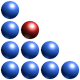# On the Extraordinary Importance of ComplexityOf all physical quantities, energy is probably the most important. Energy expresses the capacity of a body or a system to perform work. Nature works by using energy to transform matter. This is done via processes (physical, chemical, etc.). However, in order to realize these processes it is necessary to have information. Energy on its own is not sufficient. One must know what to do with it and how to do it. This is where information comes into the picture. Information is stored and delivered in a variety of ways. The DNA, for example, encodes biological information.

Information is measured in bits. Shannon’s Information Theory states that entropy is a measure of information:Entropy, however, has many facets. While it measures the amount of information necessary in order to describe a system, it also quantifies the amount of disorder contained therein. The above equation is of course for a single variable (dimension). When more dimensions are involved, structure emerges. This is because of correlations, or interdependencies, that form. Examples of interdependencies are depicted below:By the way, conventional linear correlations cannot be used in situations like the ones above. In fact, in order to deal with complex and intricate structure in data we have devised a generalized correlation scheme based on quantum physics and neurology. But that is another story.

An example of structure (in 29 dimensions) is shown below. It has the form of a map. The black dots represent the said interdependencies. In general, the map’s structure changes in time.In Nature we witness the interplay of two opposed forces: the incessant urge to create structure (using entropy, i.e. information) and the persistent compulsion to destroy it (turning it into entropy, i.e. disorder). This is represented by the following equation which is also a formal definition of complexity.In the above equation S stands for structure while E represents entropy. An example of the spontaneous emergence of structure is that of protein folding from a set of amino acids:Other examples of emergence of structure are biospheres, societies, or galaxies. An example of structure destruction (structure to entropy transformation over time) is:This brings us to the key point of this blog. Complexity not only captures and quantifies the intensity of the dynamic interaction between Structure and Entropy, it also measures the amount of information that is the result of structure. In fact, entropy – the ‘E’ in the complexity equation – is already a measure of information. However, the ‘S’ holds additional information which is ‘contained’ in the structure of the interdependency map (known also as complexity map). In other words, S encodes additional information to that provided by the Shannon equation. In the example in 29 dimensions shown above the information breakdown is as follows:

• Shannon’s information  = 60.25 bits
• Information due to structure = 284.80 bits
• Total information = 345.05 bits

In this example, structure furnishes nearly five times more information than the sum of the information content of each dimension. For higher dimensions the ratio can be significantly higher.

Complexity is not just a measure of how intricate, or sophisticated, something is. It has a deeper significance in that it defines the topology of information flow and, therefore, of the processes which make Nature work.

www.ontonix.com